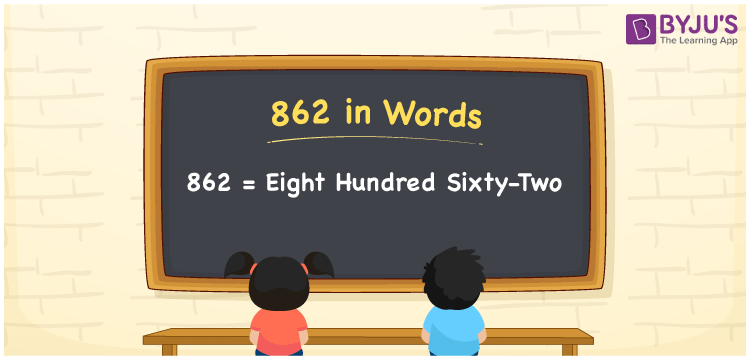# 862 in Words

We can write 862 in words as Eight hundred sixty-two. If you purchased 862 table pins, then you can say, “I purchased Eight hundred sixty-two table pins”. Thus, we use the word form or number name of 862 to convey the numeral to others. In this article, you will learn how to convert the cardinal number 862 into words in an easily understandable method.

 862 in words Eight hundred sixty-two Eight hundred sixty-two in Numbers 862

## 862 in English Words

We generally use the English alphabet to express numbers in words. So, we spell 862 in English words as “Eight hundred sixty-two”.## How to Write 862 in Words?

The number 862 contains three digits, so let’s create a place value chart to define the respective place values for these digits.

 Hundreds Tens Ones 8 6 2

Here, ones = 2, tens = 6, hundreds = 8

Let us write the expanded form of these numbers.

That means, 8 × Hundred + 6 × Ten + 2 × One

= 8 × 100 + 6 × 10 + 2 × 1

= 800 + 60 + 2

= Eight hundred + Sixty + Two

= Eight hundred sixty-two

Therefore, 862 in words is written as Eight hundred sixty-two.

Learn in detail about place value here.

862 is a natural number that precedes 863 and succeeds 861.

862 in words – Eight hundred sixty-two

Is 862 an even number? – Yes

Is 862 an odd number? – No

Is 862 a prime number? – No

Is 862 a composite number? – Yes

Is 862 a perfect square number? – No

Is 862 a perfect cube number? – No

## Frequently Asked Questions on 862 in Words

Q1

### How do you write 862 in words?

The number 862 can be written in words as “Eight hundred sixty-two”.
Q2

### Write 862.50 in words.

We can write the decimal number 862.50 in words as: 862.50 = Eight hundred sixty-two and five-tenths (or) 862.50 = Eight hundred sixty-two point five zero
Q3

### How to write Rs. 862 in words on a cheque?

On a cheque, we generally write Rs. 862 in words as “Eight hundred sixty-two rupees only”.